FAIL (the browser should render some flash content, not this).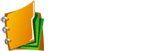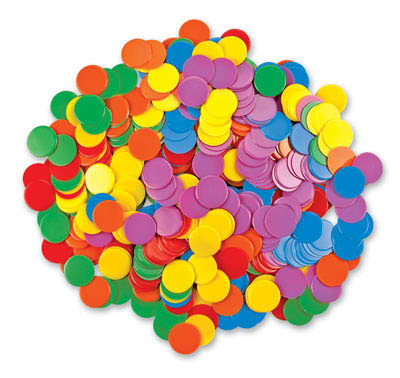PPT

• Pre

• 1.1

• 1.1b

• 1.2
• 1.2c

• 1.3

• 1.3b

• 1.4

• 1.5
• 1.6
• 1.6p2

Number Sense: Multiplying and Dividing Integers

In this section, students will learn:

• to demonstrate the multiplication and division of integers, concretely, pictorially, and symbolically

• We will also look into different strategies with multiplying integers with two digits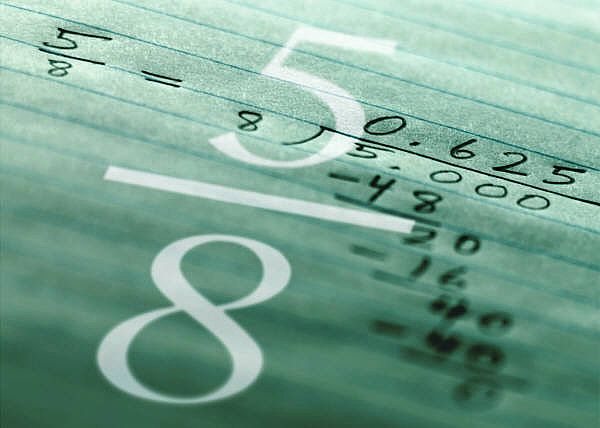PPT

• 2a
• 2b
• 2.1
• 2.2
• 2.3
• 2.4
• 2.5
• 2.6
Fractions and BEDMAS

In this section, students will learn:

• to mutliply and divide fractions and mixed numbers, concretely, pictorially, and symbolicallyMath 8 Principles Ch 3

•  Section 3.2b Common Fractions as Percentages
•  Section 3.3 Evaluating the Percent of an Integer
•  Section 3.3b Finding the Number with Percentage
•  Section 3.4 Applications of Percentages

PPT

• 3.1
• 3.2
• 3.2
• 3.3
• 3.3b
• 3.4
Percentages

In this section, students will learn:

• to convert percentages to decimals and fractions
• to represent percentages between 0% and 100% concretely, pictorially, and symbolically
• to solve problems involving percentages and combined percentagesMath 8 Principles Ch 4

PPT

• 4.1
• 4.2
• 4.3
• 4.4

Rates and Ratios

In this chapter, students will learn:

• to demonstrate an understanding of ratios and rates
• to solve problems involing rates, ratios, and proportional reasoning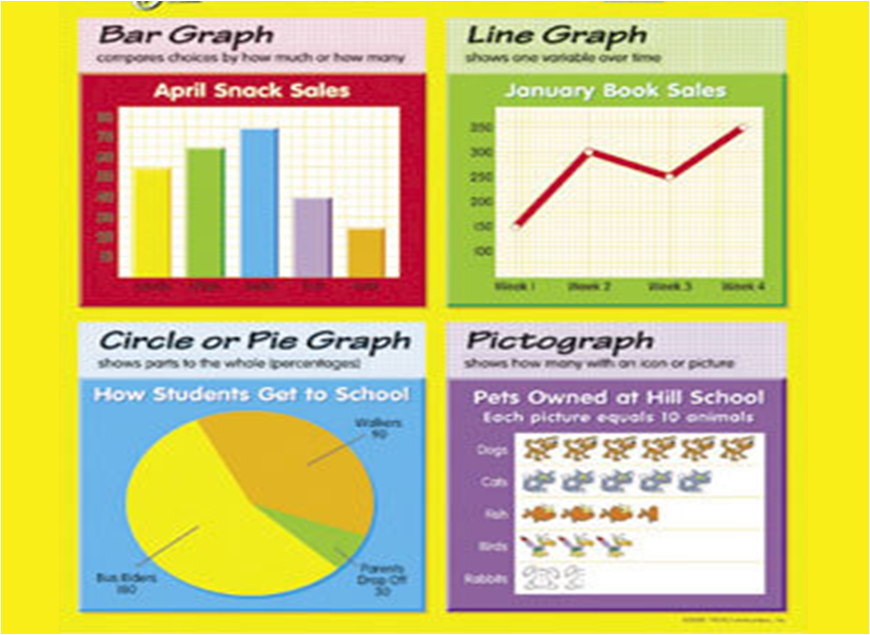Math 8 Principles Ch 5

• 5.1
• 5.1b
• 5.2
• 5.3
• 5.4

In this section, students will learn:

• to critique ways in which data is presented
• to identify how information could be misrepresented using graphs
• to present data using appropriate graphsMath 8 Principles Ch 6

• 6.1
• 6.2
• 6.3
• 6.4

Probability

In this section, students will learn:

• to solve problems involving probability and independent events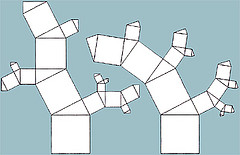Math 8 Principles Ch 7
PPT

• 7.1
• 7.2
• 7.3
• 7.4
• 7.5

Pythagorean Properties

In this chapter, students will learn:

• to develop and apply the Pythagorean Theorem
• to approximate square roots that are not perfect squares
• to display an understanding of perfect squares and squares roots of whole numbers, concretely, pictorially, and symbolically
• to solve problems by applying the Pythagoreran TheoremMath 8 Principles Ch 8
Video

PPT

• 8.1
• 8.2
• 8.3a
• 8.3
• 8.4
• C8R
• Stair
• Rotate
Surface Areas of 3D Solids

In this section, students will learn:

• to construct top, front, and side views for 3D objects
• to draw next for 3D solids
• to determine the surface areas of prisms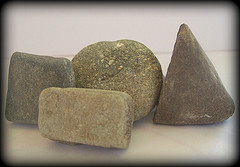Math 8 Principles Ch 9
Video
PPT

• 9.1
• 9.2
• 9.3
• 9.4
• C9R

Volume of 3D Solids

In this section, students will learn:

• to develop and apply formulas for determining the volume of prisms and cylinders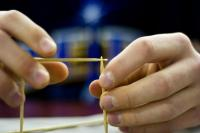Math 8 Principles Ch 10
PPT

• 10.1

• 10.2

• 10.3

• 10R

Linear Relations

In this section, students will learn:

• to graph and analyse two variable linear equations
• to display an understanding of linear equation, concretely, pictorially, and symbolically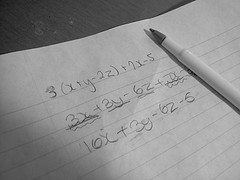Math 8 Principles Ch 11

• 11.1

• 11.2

• 11.3

• 11.4

Solving Linear Equations

In this section, students will learn:

• Desmos Parking Game
• to model and solve problems using linear equations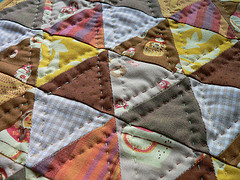Math 8 Principles Ch 12

•  Section 12.1 Exploring Tesselations
•  Section 12.2 Constructing Tesselations

•  Section 12.3 Constructing Tesselations (Part 2)

• 12.1
• 12.2

• 12.3

Tesselations

In this section, students will learn:

• to create tesslations
• to explain which shapes can be used to create tesseltations
• identify tesselations in the environment